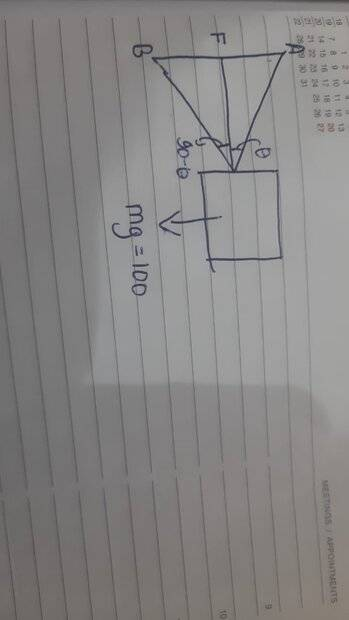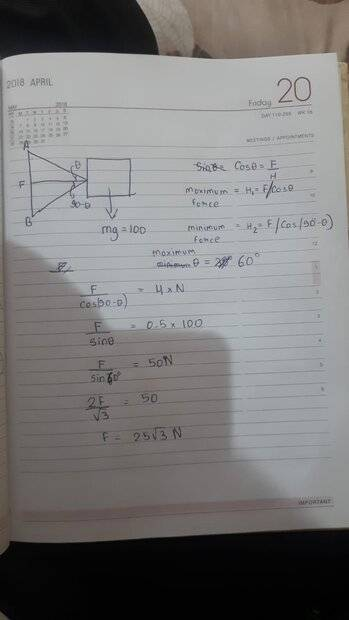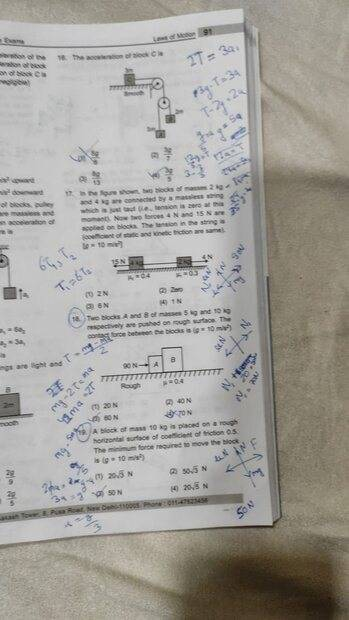# Calculate the minimum force required

misconception... to think that the block will always move when there is a force that is greater than the friction.

Homework Statement
A block of mass 10kg is placed on a rough horizontal surface with a coefficient of friction of 0.5. the minimum force required to move the block is.(g=10 m/s*s)
Relevant Equations
coefficint of friction * normal reaction = friction force
I tried taking the components but got stuck there on what value of theta I should takeWhat's ##\theta##?

PeroK said:What's ##\theta##?
that's the angle
like sin30 here 30 is theta

that's the angle
What angle?

PeroK said:
What angle?
the angle on which the force is acting on the block with respect to horizontal

the angle on which the force is acting on the block with respect to horizontal
Ah, okay. How far did you get in analysing the forces? Can you post where you got stuck?

well I drew this diagram firstthen for friction to be minimum I took b as my direction force acting on block
then wrote the equations
Fcosθ+N=mg------(1)
Fsinθ-friction of coefficient *N=ma-------(2)

now I don't know what to do after this

well I drew this diagram first View attachment 316634
then for friction to be minimum I took b as my direction force acting on block
then wrote the equations
Fcosθ+N=mg------(1)
Fsinθ-friction of coefficient *N=ma-------(2)

now I don't know what to do after this
You need to put an arrow on forces in your diagram.

Do you think you should pull the block above the horizontal or below the horizontal?

I don't understand the point of A and B.

PeroK said:
You need to put an arrow on forces in your diagram.

Do you think you should pull the block above the horizontal or below the horizontal?

I don't understand the point of A and B.
actually, ignore them
just think that te force applied on the block Is in the direction of B

actually, ignore them
just think that te force applied on the block Is in the direction of B
Are you sure about that? You want to pull the block into the ground? Isn't that going to increase friction and make it harder to move?

its going to pull the block off the ground.

can you just solve it on a piece of paper and post it
it would be very kind of you.can you just solve it on a piece of paper and post it
it would be very kind of you.It would also be cheating! We don't do that here:

https://www.physicsforums.com/help/homeworkhelp/

its going to pull the block off the ground.
am i wrong?

You do not know ##\theta##. However, for every value of ##\theta## there will be a corresponding necessary force. The minimum force required will be the smallest of those forces.

Orodruin said:
You do not know ##\theta##. However, for every value of ##\theta## there will be a corresponding necessary force. The minimum force required will be the smallest of those forces.
yeah i understand it

sir PeroK
help!

nailed it mansir PeroK
help!
Your making a fundamental mistake by pulling the block into the ground. That said, if you set up your equations to allow ##\theta## to be positive or negative, then the solution would show that minumim force involves pulling the block at an angle above the horizontal.

My advice is to redraw your diagram with ##\theta## above the horizontal.

The other point is that the minimum force to move the block involves zero accleration. I.e. when the horizontal force on the block equals the frictional force.

•PeroK said:
Your making a fundamental mistake by pulling the block into the ground. That said, if you set up your equations to allow ##\theta## to be positive or negative, then the solution would show that minumim force involves pulling the block at an angle above the horizontal.

My advice is to redraw your diagram with ##\theta## above the horizontal.

The other point is that the minimum force to move the block involves zero accleration. I.e. when the horizontal force on the block equals the frictional force.
if i take acceleration to be zero I would give me the force that would not move the block
to make a block move it need to have some acceleration

PeroK said:
Your making a fundamental mistake by pulling the block into the ground. That said, if you set up your equations to allow ##\theta## to be positive or negative, then the solution would show that minumim force involves pulling the block at an angle above the horizontal.

My advice is to redraw your diagram with ##\theta## above the horizontal.

The other point is that the minimum force to move the block involves zero accleration. I.e. when the horizontal force on the block equals the frictional force.
yeah got your point the θ must be above the horizontal.
thank you

if i take acceleration to be zero I would give me the force that would not move the block
to make a block move it need to have some acceleration
With the minimum force the acceleration is negligible. Above that minimum the block will move and below it the block won't move. It's a common idea.

PeroK said:
With the minimum force the acceleration is negligible. Above that minimum the block will move and below it the block won't move. It's a common idea.
considering small acceleration to be negligible we can say that

nailed it man

View attachment 316635
I don't see how you got that answer, but it doesn't look right.

Generally, you need calculus to minimise something in a problem with a continuous variable.

PeroK said:
Generally, you need calculus to minimise something in a problem with a continuous variable.
it is a basic level question of my coaching institute's daily practice paper intended for the preparation of JEE
so I think it is ought to be a little easy(not including problems with continuous variable)

it is a basic level question of my coaching institute's daily practice paper intended for the preparation of JEE
so I think it is ought to be a little easy(not including problems with continuous variable)
you are guiding me the question with understanding of high level physics
and I don't think it applies to these questions

still I am open to learn new things

it is a basic level question of my coaching institute's daily practice paper intended for the preparation of JEE
so I think it is ought to be a little easy(not including problems with continuous variable)
Then, perhaps the answer is just ##50 N##? Which is the minumum horizontal force to overcome friction. Considering a force applied at an angle is definitely a more advanced problem.

Last edited:
this
PeroK said:
Then, perhaps the answer is just ##25 N##? Which is the minumum horizontal force to overcome friction. Considering a force applied at an angle is definitely a more advanced problem.
this question has option sgiven as follows
a-20√3N
b-50√3N
c-50N
d-20√5 N

this

this question has option sgiven as follows
a-20√3N
b-50√3N
c-50N
d-20√5 N
Okay, the answer is one of those, but not a).

THE ANSWER GIVEN IS 20√5 maybe by considering that it is close to 25 as it was asked to be minimum